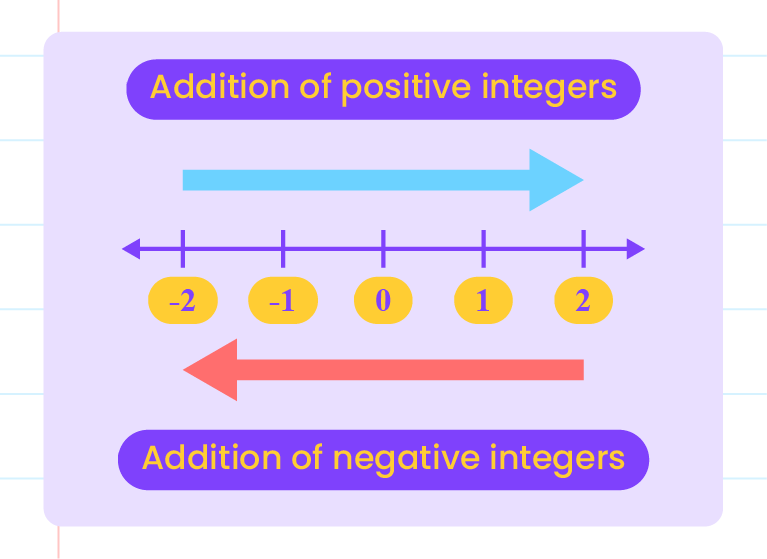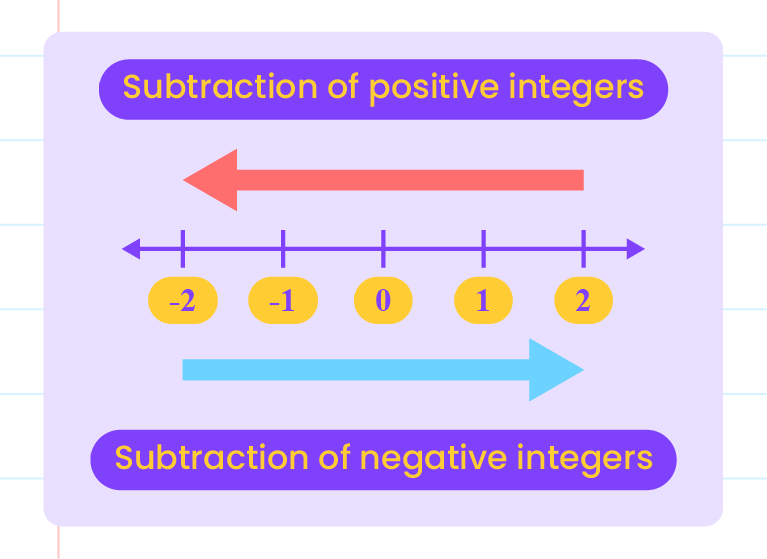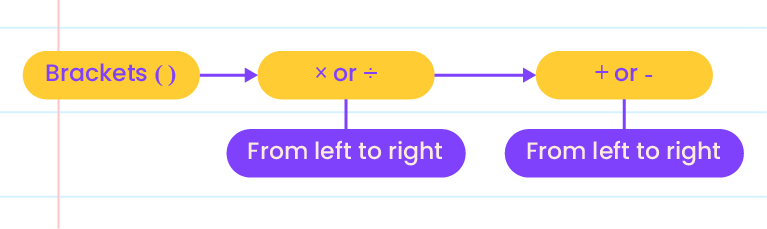## Basic Arithmetic Operations Involving Integers

1.2  Basic Arithmetic Operations Involving Integers

• Positive integers is represented by moving towards the right.
• Negative integers is represented by moving towards the left.Subtraction of integers:

• Positive integers is represented by moving towards the left.
• Negative integers is represented by moving towards the right.Example Solve: (i) \begin{aligned}6-(-7)&=6+7 \\\\&=13. \end{aligned} (ii) \begin{aligned}-21+(3)&=-21+3 \\\\&=-18. \end{aligned}

Multiplication of integers:

 Operation Sign of the product $$(+)\times(+)$$ $$+$$ $$(+)\times(-)$$ $$-$$ $$(-)\times(+)$$ $$-$$ $$(-)\times(-)$$ $$+$$

Division of integers:

 Operation Sign of the quotient $$(+)\div(+)$$ $$+$$ $$(+)\div(-)$$ $$-$$ $$(-)\div(+)$$ $$-$$ $$(-)\div(-)$$ $$+$$

In general,

• The product or quotient of two integers with the same signs is a positive integer.
• The product or quotient of two integers with different signs is a negative integer.

 Example Calculate: (i) \begin{aligned}9\times(-11)&=-(9\times11) \\\\&=-99. \end{aligned} (ii) \begin{aligned}-48\div(-8)&=+(48\div8) \\\\&=6 \end{aligned}

Combined basic arithmetic operations of integer:Example Solve: (i) \begin{aligned}&\space49\div(-8+1)\\\\&=49\div(-7) \\\\&=-7. \end{aligned} (ii) \begin{aligned}&\space\dfrac{22+(-4)}{-7-2} \\\\&= \dfrac{22-4}{-9}\\\\ &=\dfrac{18}{-9}\\ \\&=-2. \end{aligned}

Laws of arithmetic operations:

Commutative Law

\begin{aligned} a+b&=b+a \\\\a\times b&=b\times a \end{aligned}

Associative Law

\begin{aligned} (a+b)+c&=a+(b+c) \\\\(a\times b)\times c&=a\times(b\times c) \end{aligned}

Distributive Law

\begin{aligned} a\times(b+c)&=a\times b+a\times c \\\\a\times(b-c)&=a\times b-a\times c \end{aligned}

Identity Law

\begin{aligned} a+0&=a \\\\a\times 0&=0 \\\\a\times 1&=a \\\\a+(-a)&=0 \\\\a\times\dfrac{1}{a}&=1 \end{aligned}